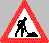CS486: Applied Logic
 Cornell University, Spring 2003

Summary

Logic is concerned with propositions and proofs. A proposition is an abstract mathematical object corresponding to a declarative sentence. We speak of the sense of a proposition, i.e. the thought expressed by it, and of its truth value.
Logic deals with the truth value of propositions independently of subject matter. The raw data of logic are representations of the abstract notion of a proposition. The truth of a proposition depends on its meaning or its sense. Access to truth is via evidence, particularly in the form of proof.
The notion of proof is used also as the basis for meaning; to understand a proposition is to know how to prove it - although one may not know whether a proof can be found. These are the basic concepts of logic and the course presents precise versions of these concepts and the relationships among them.
Computer scientists have shown how to implement the computational core of logics as a programming language and reasoning system combined into one language. Such mathematical systems form a rich class of programming logics which have proven useful in computer science. Conversely, advances in computer science have created a new field of mathematics called Formalized Mathematics. This course will demonstrate these connections between computer science and logic.

CS 486 aims at third or fourth year undergraduates and first year graduate students interested in logic.

 Notes from the current course Lecture 1: What is Logic? Handwritten notes only Part I: The Tableau Method for Propositional Logic Lecture 2: Propositional formulas Handwritten notes only Lecture 3: Valuations and Truth Handwritten notes only Lecture 4: Analytic Tableaux PS PDF Lecture 5: Consistency PS PDF Lecture 6: Completeness PS PDF Lecture 7: Compactness (Analytic Proofs) PS PDF Lecture 8: Compactness (Lindenbaum's construction) PS PDF Part II: Alternative Proof Methods Lecture 9: From Analytic Tableaux to Gentzen Systems PS PDF Lecture 10: From Gentzen Systems to Refinement Logic PS PDF Lecture 11: Refinement Logic PS PDF Lecture 12: Correctness and Completeness of Refinement Logic PS PDF Part III: First-Order Logic Lecture 13: Second Order Propositional Logic (Syntax, Substitution) PS PDF Lecture 14: Second Order Propositional Logic (Semantics and Proof Rules) PS PDF Lecture 15: First-Order Logic (Syntax and Semanctics) Handwritten notes only Lecture 16: First-Order Tableaux (Proof System) Handwritten notes only Lecture 17: First-Order Tableaux (Correctness) PS PDF Lecture 18: First-Order Tableaux (Completeness and Compactness) PS PDF Part IV: Expressing Mathematical Concepts in First-Order Logic Lecture 19: Functions and Equality PS PDF Lecture 20: Algebraic Structures PS PDF Lecture 21: Axiomatizing Integer Arithmetic PS PDF Lecture 22: Arithmetic Representability PS PDF Lecture 23: Unsolvable Problems in Logic I (Undefinability of truth and arithmetical incompleteness) PS PDF Lecture 24: Unsolvable Problems in Logic II (The theory Q and the undecidability of logic) PS PDF Lecture 25: Lambda-PRL (Formal Language and proof calculus) PS PDF Lecture 26: Proof Automation in Lambda-PRL (Tactics and Decision Procedures) PS PDF

This page is still under construction.